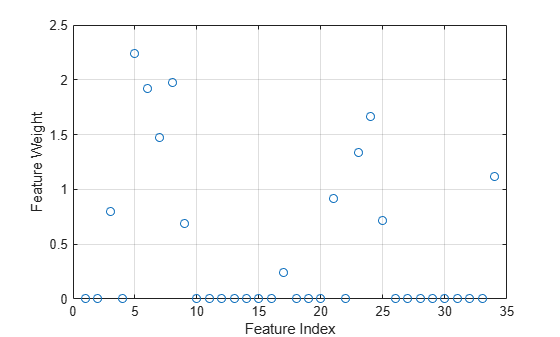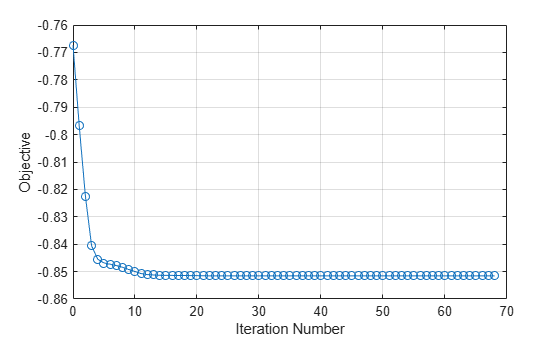# FeatureSelectionNCAClassification class

Feature selection for classification using neighborhood component analysis (NCA)

## Description

`FeatureSelectionNCAClassification` object contains the data, fitting information, feature weights, and other parameters of a neighborhood component analysis (NCA) model. `fscnca` learns the feature weights using a diagonal adaptation of NCA and returns an instance of a `FeatureSelectionNCAClassification` object. The function achieves feature selection by regularizing the feature weights.

## Construction

Create a `FeatureSelectionNCAClassification` object using `fscnca`.

## Properties

expand all

Number of observations in the training data (`X` and `Y`) after removing `NaN` or `Inf` values, stored as a scalar.

Data Types: `double`

Model parameters used for training the model, stored as a structure.

You can access the fields of `ModelParameters` using dot notation.

For example, for a FeatureSelectionNCAClassification object named `mdl`, you can access the `LossFunction` value using `mdl.ModelParameters.LossFunction`.

Data Types: `struct`

Regularization parameter used for training this model, stored as a scalar. For n observations, the best `Lambda` value that minimizes the generalization error of the NCA model is expected to be a multiple of 1/n.

Data Types: `double`

Name of the fitting method used to fit this model, stored as one of the following:

• `'exact'` — Perform fitting using all of the data.

• `'none'` — No fitting. Use this option to evaluate the generalization error of the NCA model using the initial feature weights supplied in the call to `fscnca`.

• `'average'` — Divide the data into partitions (subsets), fit each partition using the `exact` method, and return the average of the feature weights. You can specify the number of partitions using the `NumPartitions` name-value pair argument.

Name of the solver used to fit this model, stored as one of the following:

• `'lbfgs'` — Limited memory Broyden-Fletcher-Goldfarb-Shanno (LBFGS) algorithm

• `'sgd'` — Stochastic gradient descent (SGD) algorithm

• `'minibatch-lbfgs'` — stochastic gradient descent with LBFGS algorithm applied to mini-batches

Relative convergence tolerance on the gradient norm for the `'lbfgs'` and `'minibatch-lbfgs'` solvers, stored as a positive scalar value.

Data Types: `double`

Maximum number of iterations for optimization, stored as a positive integer value.

Data Types: `double`

Maximum number of passes for `'sgd'` and `'minibatch-lbfgs'` solvers. Every pass processes all of the observations in the data.

Data Types: `double`

Initial learning rate for the `'sgd'` and `'minibatch-lbfgs'` solvers, stored as a positive real scalar. The learning rate decays over iterations starting at the value specified for `InitialLearningRate`.

Use the `NumTuningIterations` and `TuningSubsetSize` name-value pair arguments to control the automatic tuning of initial learning rate in the call to `fscnca`.

Data Types: `double`

Verbosity level indicator, stored as a nonnegative integer. Possible values are:

• 0 — No convergence summary

• 1 — Convergence summary, including norm of gradient and objective function value

• >1 — More convergence information, depending on the fitting algorithm. When you use the `'minibatch-lbfgs'` solver and verbosity level > 1, the convergence information includes the iteration log from intermediate minibatch LBFGS fits.

Data Types: `double`

Initial feature weights, stored as a p-by-1 vector of positive real scalars, where p is the number of predictors in `X`.

Data Types: `double`

Feature weights, stored as a p-by-1 vector of real scalars, where p is the number of predictors in `X`.

If `FitMethod` is `'average'`, then `FeatureWeights` is a p-by-m matrix. m is the number of partitions specified via the `'NumPartitions'` name-value pair argument in the call to `fscnca`.

The absolute value of `FeatureWeights(k)` is a measure of the importance of predictor `k`. A `FeatureWeights(k)` value that is close to 0 indicates that predictor `k` does not influence the response in `Y`.

Data Types: `double`

Fit information, stored as a structure with the following fields.

Field NameMeaning
`Iteration`Iteration index
`Objective`Regularized objective function for minimization
`UnregularizedObjective`Unregularized objective function for minimization
`Gradient`Gradient of regularized objective function for minimization
• For classification, `UnregularizedObjective` represents the negative of the leave-one-out accuracy of the NCA classifier on the training data.

• For regression, `UnregularizedObjective` represents the leave-one-out loss between the true response and the predicted response when using the NCA regression model.

• For the `'lbfgs'` solver, `Gradient` is the final gradient. For the `'sgd'` and `'minibatch-lbfgs'` solvers, `Gradient` is the final mini-batch gradient.

• If `FitMethod` is `'average'`, then `FitInfo` is an m-by-1 structure array, where m is the number of partitions specified via the `'NumPartitions'` name-value pair argument.

You can access the fields of `FitInfo` using dot notation. For example, for a FeatureSelectionNCAClassificationobject named `mdl`, you can access the `Objective` field using `mdl.FitInfo.Objective`.

Data Types: `struct`

Predictor means, stored as a p-by-1 vector for standardized training data. In this case, the `predict` method centers predictor matrix `X` by subtracting the respective element of `Mu` from every column.

If data is not standardized during training, then `Mu` is empty.

Data Types: `double`

Predictor standard deviations, stored as a p-by-1 vector for standardized training data. In this case, the `predict` method scales predictor matrix `X` by dividing every column by the respective element of `Sigma` after centering the data using `Mu`.

If data is not standardized during training, then `Sigma` is empty.

Data Types: `double`

Predictor values used to train this model, stored as an n-by-p matrix. n is the number of observations and p is the number of predictor variables in the training data.

Data Types: `double`

Response values used to train this model, stored as a numeric vector of size n, where n is the number of observations.

Data Types: `double`

Observation weights used to train this model, stored as a numeric vector of size n. The sum of observation weights is n.

Data Types: `double`

## Methods

 loss Evaluate accuracy of learned feature weights on test data predict Predict responses using neighborhood component analysis (NCA) classifier refit Refit neighborhood component analysis (NCA) model for classification

## Examples

collapse all

`load ionosphere`

The data set has 34 continuous predictors. The response variable is the radar returns, labeled as b (bad) or g (good).

Fit a neighborhood component analysis (NCA) model for classification to detect the relevant features.

`mdl = fscnca(X,Y);`

The returned NCA model, `mdl`, is a `FeatureSelectionNCAClassification` object. This object stores information about the training data, model, and optimization. You can access the object properties, such as the feature weights, using dot notation.

Plot the feature weights.

```figure() plot(mdl.FeatureWeights,'ro') xlabel('Feature Index') ylabel('Feature Weight') grid on```The weights of the irrelevant features are zero. The `'Verbose',1` option in the call to `fscnca` displays the optimization information on the command line. You can also visualize the optimization process by plotting the objective function versus the iteration number.

```figure plot(mdl.FitInfo.Iteration,mdl.FitInfo.Objective,'ro-') grid on xlabel('Iteration Number') ylabel('Objective')```The `ModelParameters` property is a `struct` that contains more information about the model. You can access the fields of this property using dot notation. For example, see if the data was standardized or not.

`mdl.ModelParameters.Standardize`
```ans = logical 0 ```

`0` means that the data was not standardized before fitting the NCA model. You can standardize the predictors when they are on very different scales using the `'Standardize',1` name-value pair argument in the call to `fscnca` .

## Copy Semantics

Value. To learn how value classes affect copy operations, see Copying Objects (MATLAB).